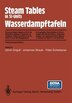Steam Tables in SI-Units / Wasserdampftafeln: Concise Steam Tables in SI-Units (Student's Tables…

EditorUlrich Grigull, Johannes Straub, Peter Peter Schiebener

Pricing and Purchase Info

$98.59 online$110.50 list price save 10%
Earn 493 plum® points

Prices and offers may vary in store

Quantity:

In stock online

Ships free on orders over \$25

Not available in stores

This booklet is mainly meant for students at universities and colleges to solve problems in the field of power and chemical engineering, where water and steam are serving as working or process medium. Tables and diagrams will support engineers in research work and industrial practice too. All tabulated values given were recalculated; the thermodynamical properties have been calculated according to the 1984 IAPS formulation, the remaining properties result from IAPS`s current releases. The increments for temperature and pressure for the saturation tables were decreased. In addition ten properties were added. Three new h,s-diagrams for compressed water will be useful in geographical and in jet cutting applications.
Title:Steam Tables in SI-Units / Wasserdampftafeln: Concise Steam Tables in SI-Units (Student's Tables…Format:PaperbackPublished:December 28, 1989Publisher:Springer Berlin HeidelbergLanguage:English

The following ISBNs are associated with this title:

ISBN - 10:3540518886

ISBN - 13:9783540518884

Reviews

I. Quantities, Constants, Units.- Table A. Quantities and Units Presented.- References for Table A.- Table B. Constant Quantities of Ordinary Water.- Table C. Conversion of Units.- C 1. Pressure.- C 2. Temperature.- C 3. Energy, Work, Quantity of Heat.- C 4. Specific Heat Capacity, Specific Entropy, Specific Gas Constant.- C 5. Thermal Conductivity.- C 6. Viscosity.- C 7. Thermal Diffusivity, Kinematic Viscosity, Diffusion Coefficient.- C 8. Surface Tension.- Table D. Thermodynamic Relationships between the Properties p, ?, T, s, u, h and their Derivatives.- II. Tables of the Properties of Ordinary Water Substance.- Table 1. Thermodynamic and Transport Properties of Liquid Water and Superheated Steam inclusive the Saturation Values from the Triple Point to 1000°C and 100 MPa: p, t, ?, h, u, s, cp, ?p, ?, ? (Pressure Table).- Table 2. Thermodynamic and Transport Properties of Saturated Water and Steam.- 1. ?, h, r, w, s, cp, cv (Temperature Table).- 2. ?, ?, v,r, ?, b, ?y, ?s (Temperature Table).- 3. $$\alpha _p ,\:\delta _h ,\:\delta _T ,\:\frac{{dr}}{{dT}},\frac{{dp}}{{dT}}\left| {_{sat} ,\frac{{d^2 p}}{{dT^2 }}} \right|_{sat} ,c_{sat} ,c,\chi _T$$ (Temperature Table).- 4. ?, h, r, u, s, cp, cv (Pressure Table).- 5. ?, ?, v, a, Pr, ?, b, ?y, ?s (Pressure Table).- 6. $$\alpha _p ,\:\delta _h ,\:\delta _T ,\:\frac{{dr}}{{dT}},\frac{{dp}}{{dT}}\left| {_{sat} ,\frac{{d^2 p}}{{dT^2 }}} \right|_{sat} ,c_{sat} ,c,\chi _T$$(Pressure Table).- Table 3. Properties of Water at 0.1 MPa from -30 °C to the Saturation Temperature.- III. Diagrams.- Fig. 1. Dynamic Viscosity ? in the p, T-Plane.- Fig.2. Thermal Conductivity ? in the p, T-Plane.- Fig.3. Prandtl-Number Pr = ?cp / ? in the p, T-Plane.- Fig.4. Pressure-Enthalpy (log10p, h)-Diagram.- Fig. 5. T, s, p-Surface of Water Substance.- Fig. 6. T, s-Diagram.- Fig. 7. p, ?, T-Surface of Water Substance.- Fig. 8. Mollier h, s-Diagram for Steam p ? 100MPa; 50°C ? t ? 800°C.- Fig.9. Mollier h, s-Diagram for Compressed Water p ? 150MPa; -2°C ? t ? 20°C.- Fig. 10. Mollier h, s-Diagram for Compressed Water p ? 1100 MPa; -22°C ? t ? 72°C.- Fig. 11. Mollier h, s-Diagram for Compressed Water p ? 2900MPa; -22°C ? t ? 420°C.